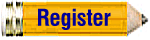Virginia Algebra II and Trigonometry
Create worksheets, tests, and quizzes for Virginia Algebra II and Trigonometry.User Name: Password: Worksheet Title: Practice Problems Test Quiz SOL Review Midterm Final Exam or fill in: To keep server load down, there is a maximum of 100 questions per worksheet. Create Answer Sheet (Pop-Up Window) Show how to solve it! (Pop-Up Window) Mix up the problems. (Good for tests) Multiple Choice (Good for Standardized Test practice) Move Instructions into Problems Interactive Online Test (students only! Limited to 20 questions at a time) Answer Blanks: Boxes Blanks Percent of problems with fractional coefficients 0 5 10 20 30 40 50 60 70 80 90 100 (Only where applicable) Start Numbering with: (Whole Numbers only) Save this EasyFramework (Teacher Plus+ Account Only!) as a HomeworkTestQuizOther Condense - Leave no room for student work Select the number of each type of objective:(Selecting Random will randomly generate all subtypes)Click on any title to see the free sample worksheet. (only the first few samples are free) (Random) AIIT.1a Rational Expressions a. Multiplying Rational Expressions (Easier) (Requires Factoring!) b. Multiplying Rational Expressions (Harder) (Requires Factoring!) c. Dividing Rational Expressions (Easier) (Requires Factoring!) d. Dividing Rational Expressions (Harder) (Requires Factoring!) e. Adding Rational Expressions(Easier) (Requires Factoring!) f. Adding Rational Expressions (Harder) (Requires Factoring!) g. Subtracting Rational Expressions(Easier) (Requires Factoring!) h. Subtracting Rational Expressions (Harder) (Requires Factoring!) i. Mixed Rational Expressions (Multiple Operations with simplify)(Easier) (Requires Factoring!) j. Mixed Rational Expressions (Multiple Operations with simplify) (Harder) (Requires Factoring!) (Random) AIIT.1b Radical Expressions (Random) AIIT.1c Radical And Rational (Random) AIIT.1d Factoring Polynomials (Random) AIIT.2 Sequences and Series (Random) AIIT.3 Complex Numbers (Random) AIIT.4a Absolute Value (Random) AIIT.4b Quadratic Equations (Random) AIIT.4cd Rationals and Radical Equations (Random) AIIT.5 NonLinear Systems (Random) AIIT.6 Transformations of Functions (Random) AIIT.7 Analyzing Functions (Random) AIIT.8 Solutions, Zeros, and X-Intercepts (Random) AIIT.9 Curve of Best Fit (Random) AIIT.10 Variation (Random) AIIT.11 Normal Distributions (Random) AIIT.12 Permutations and Combinations (Random) AIIT.13 Trigonometric Definitions (Random) AIIT.14 Relating Trigonometric Functions (Random) AIIT.15 Special Angles (Random) AIIT.16 Inverse Trigonometric Functions (Random) AIIT.17 Trigonometric Identities (Random) AIIT.18 Graphing Trigonometric Functions (Random) AIIT.19 Graphing Inverses (Random) AIIT.20 Trigonometric Equations (Random) AIIT.21 Law of Sines and Cosines Permissions - Can you photocopy these worksheets?